# ISEE Middle Level Math : How to find the decimal equivalent of a fraction

## Example Questions

1 2 11 12 13 14 15 16 17 19 Next →

### Example Question #181 : Fractions

What is the decimal equivalent to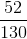?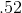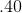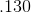Explanation:

To solve, divide the numerator (top half of the fraction) by the denominator (bottom half of the fraction):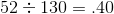Answer:### Example Question #182 : How To Find The Decimal Equivalent Of A Fraction

What is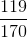written as a decimal?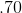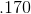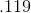Explanation:

To solve, divide the numerator (top half of the fraction) by the denominator (bottom half of the fraction):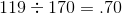Answer:### Example Question #183 : How To Find The Decimal Equivalent Of A Fraction

Convert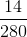to a decimal.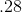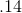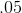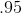Explanation:

To convert a fraction to a decimal, divide the numerator by the denominator: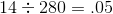Answer:### Example Question #184 : How To Find The Decimal Equivalent Of A Fraction

What is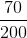as a decimal?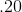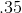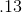Explanation:

To convert a fraction to a decimal, divide the numerator by the denominator: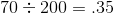Answer:### Example Question #181 : How To Find The Decimal Equivalent Of A Fraction

There are 4 children of the following heights: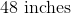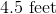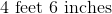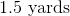How tall is the child who is not the same height as the other children?Explanation:

Given that 4.5 feet; 4 feet, six inches, and 1.5 yards are all the equivalent of 4 and-a-half feet, the child who is 48 inches is only 4 feet tall. Therefore, he is of the different height.

### Example Question #182 : How To Find The Decimal Equivalent Of A Fraction

Turn the following fraction into a decimal.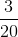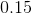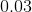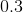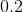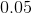Explanation:

To make a fraction into a decimal, you must first make the denominator.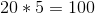so we must also multiple the numerator by.

This gives us the fraction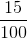.

Then all you have to do is take the numerator and place a decimal in front of it which gives you.

1 2 11 12 13 14 15 16 17 19 Next →

### All ISEE Middle Level Math Resources